Journal of Experimental and Theoretical Physics
 HOME | SEARCH | AUTHORS | HELP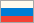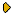Journal IssuesGolden PagesAbout This journalAims and ScopeEditorial BoardManuscript SubmissionGuidelines for AuthorsManuscript StatusContactsZhETF, Vol. 137, No. 3, p. 483 (March 2010)
(English translation - JETP, Vol. 110, No. 3, p. 426, March 2010 available online at www.springer.com )

BCS-BEC CROSSOVER AND QUANTUM HYDRODYNAMICS IN p-WAVE SUPERFLUIDS WITH A SYMMETRY OF THE A1 PHASE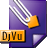DJVU (227.1K)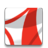PDF (606.3K)
We solve the Leggett equations for the BCS-BEC crossover in a three-dimensional resonance p-wave superfluid with the symmetry of the A1 phase. We calculate the sound velocity, the normal density, and the specific heat for the BCS domain (μ>0), for the BEC domain (μ<0), and close to the important point μ=0 in the 100 % polarized case. We find the indications of a quantum phase transition close to the point μ(T=0)=0. Deep in the BCS and BEC domains, the crossover ideas of Leggett, Nozieres, and Schmitt-Rink work quite well. We discuss the spectrum of orbital waves, the paradox of intrinsic angular momentum and the complicated problem of chiral anomaly in the BCS A1 phase at T=0. We present two different approaches to the chiral anomaly, based on supersymmetric hydrodynamics and on the formal analogy with the Dirac equation in quantum electrodynamics. We evaluate the damping of nodal fermions due to different decay processes in the superclean case at T=0 and find that a ballistic regime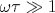occurs. We propose to use aerogel or nonmagnetic impurities to reach the hydrodynamic regime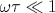at T=0. We discuss the concept of the spectral flow and exact cancelations between time derivatives of anomalous and quasiparticle currents in the equation for the total linear momentum conservation. We propose to derive and solve the kinetic equation for the nodal quasiparticles in both the hydrodynamic and ballistic regimes to demonstrate this cancelation explicitly. We briefly discuss the role of the other residual interactions different from damping and invite experimentalists to measure the spectrum and damping of orbital waves in the A phase of 3 He at low temperatures.